Practice
Resources
Contests
Online IDE
New
Free Mock
Scaler
Practice
Improve your coding skills with our resources
Contests
Compete in popular contests with top coders
Scaler
Explore Offerings by SCALER## Welcome to Interviewbit, help us create the best experience for you!

Currently, You are a:College/University *
Enter the name of your college
Branch *
Year of completion *College/University *
Enter the name of your college
Branch *
Year of completion *Current Company *
Enter company name
Experience *## You're all set!Full name *
Email *

By creating an account, I acknowledge that I have read and agree to InterviewBit’s Terms and Privacy Policy .## Welcome back!

Email *Go to Problems

Be a Code Ninja!

# Merge sort algorithm

Merge sort is one of the most efficient sorting algorithms. It works on the principle of Divide and Conquer. Merge sort repeatedly breaks down a list into several sublists until each sublist consists of a single element and merging those sublists in a manner that results into a sorted list.

## A merge sort works as follows:

### Top-down Merge Sort Implementation:

The top-down merge sort approach is the methodology which uses recursion mechanism. It starts at the Top and proceeds downwards, with each recursive turn asking the same question such as “What is required to be done to sort the array?” and having the answer as, “split the array into two, make a recursive call, and merge the results.”, until one gets to the bottom of the array-tree.

Example: Let us consider an example to understand the approach better.

1. Divide the unsorted list into n sublists, each comprising 1 element (a list of 1 element is supposed sorted).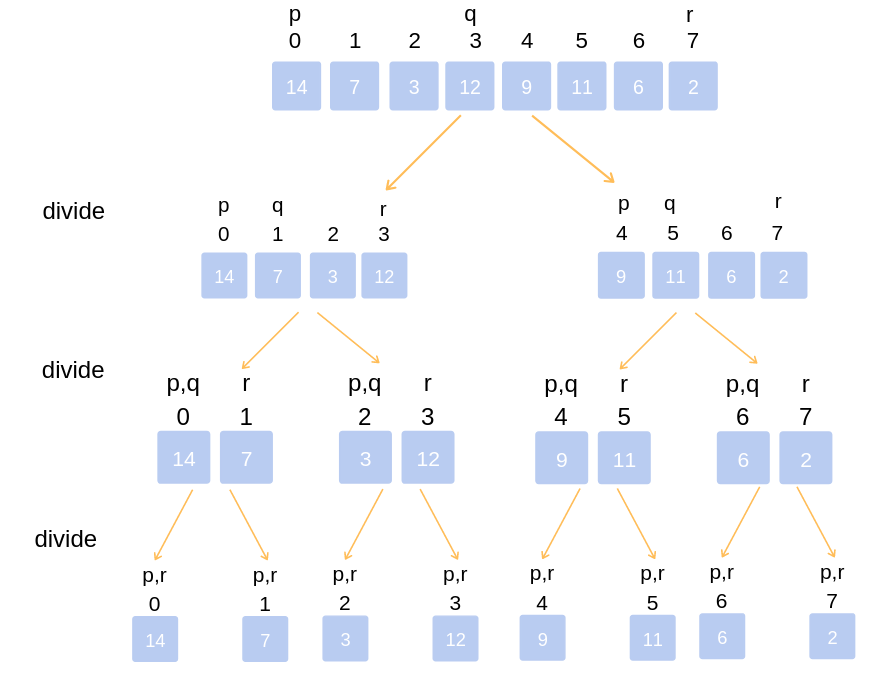Top-down Implementation

1. Repeatedly merge sublists to produce newly sorted sublists until there is only 1 sublist remaining. This will be the sorted list.

### Merging of two lists done as follows:

The first element of both lists is compared. If sorting in ascending order, the smaller element among two becomes a new element of the sorted list. This procedure is repeated until both the smaller sublists are empty and the newly combined sublist covers all the elements of both the sublists.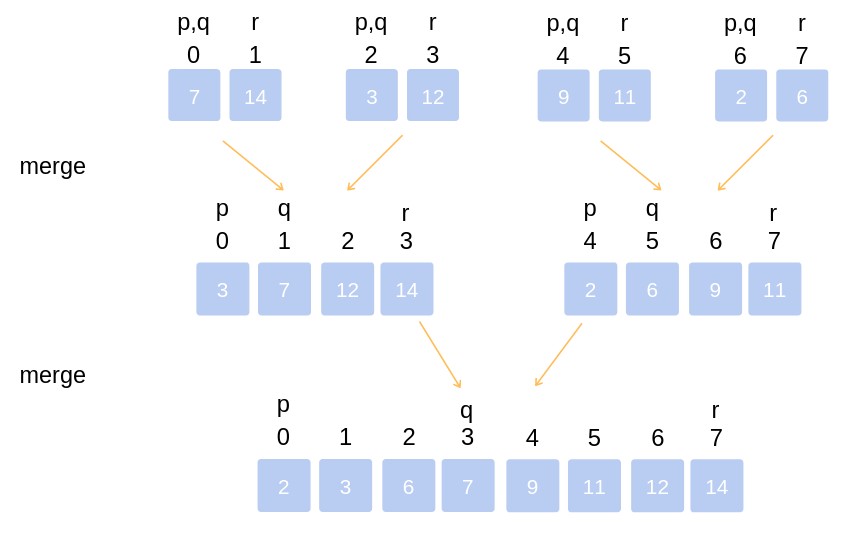Merging of two lists

## Implementation Of Merge Sort

``````// example of merge sort in C/C++
// merge function take two intervals
// one from start to mid
// second from mid+1, to end
// and merge them in sorted order

void merge(int *Arr, int start, int mid, int end) {
// create a temp array
int temp[end - start + 1];

// crawlers for both intervals and for temp
int i = start, j = mid+1, k = 0;

// traverse both arrays and in each iteration add smaller of both elements in temp
while(i <= mid && j <= end) {
if(Arr[i] <= Arr[j]) {
temp[k] = Arr[i];
k += 1; i += 1;
}
else {
temp[k] = Arr[j];
k += 1; j += 1;
}
}

// add elements left in the first interval
while(i <= mid) {
temp[k] = Arr[i];
k += 1; i += 1;
}

// add elements left in the second interval
while(j <= end) {
temp[k] = Arr[j];
k += 1; j += 1;
}

// copy temp to original interval
for(i = start; i <= end; i += 1) {
Arr[i] = temp[i - start]
}
}

// Arr is an array of integer type
// start and end are the starting and ending index of current interval of Arr

void mergeSort(int *Arr, int start, int end) {

if(start < end) {
int mid = (start + end) / 2;
mergeSort(Arr, start, mid);
mergeSort(Arr, mid+1, end);
merge(Arr, start, mid, end);
}
}``````
``````// example of merge sort in Java
// merge function take two intervals
// one from start to mid
// second from mid+1, to end
// and merge them in sorted order

void merge(int Arr[], int start, int mid, int end) {

// create a temp array
int temp[] = new int[end - start + 1];

// crawlers for both intervals and for temp
int i = start, j = mid+1, k = 0;

// traverse both arrays and in each iteration add smaller of both elements in temp
while(i <= mid && j <= end) {
if(Arr[i] <= Arr[j]) {
temp[k] = Arr[i];
k += 1; i += 1;
}
else {
temp[k] = Arr[j];
k += 1; j += 1;
}
}

// add elements left in the first interval
while(i <= mid) {
temp[k] = Arr[i];
k += 1; i += 1;
}

// add elements left in the second interval
while(j <= end) {
temp[k] = Arr[j];
k += 1; j += 1;
}

// copy temp to original interval
for(i = start; i <= end; i += 1) {
Arr[i] = temp[i - start]
}
}

// Arr is an array of integer type
// start and end are the starting and ending index of current interval of Arr

void mergeSort(int Arr[], int start, int end) {

if(start < end) {
int mid = (start + end) / 2;
mergeSort(Arr, start, mid);
mergeSort(Arr, mid+1, end);
merge(Arr, start, mid, end);
}
}``````
``````# example of merge sort in Python
# merge function take two intervals
# one from start to mid
# second from mid+1, to end
# and merge them in sorted order
def merge(Arr, start, mid, end) :

# create a temp array
temp[] =  * (end - start + 1)

# crawlers for both intervals and for temp
i, j, k = start, mid+1, 0

# traverse both lists and in each iteration add smaller of both elements in temp
while(i <= mid and j <= end) :
if(Arr[i] <= Arr[j]) :
temp[k] = Arr[i]
k += 1; i += 1
else :
temp[k] = Arr[j]
k += 1; j += 1

# add elements left in the first interval
while(i <= mid) :
temp[k] = Arr[i]
k += 1; i += 1

# add elements left in the second interval
while(j <= end) :
temp[k] = Arr[j]
k += 1; j += 1

# copy temp to original interval
for i in range (start, end+1) :
Arr[i] = temp[i - start]

# Arr is an array of integer type
# start and end are the starting and ending index of current interval of Arr

def mergeSort(Arr, start, end) {

if(start < end) :
mid = (start + end) / 2
mergeSort(Arr, start, mid)
mergeSort(Arr, mid+1, end)
merge(Arr, start, mid, end)``````

### Bottom-Up Merge Sort Implementation:

The Bottom-Up merge sort approach uses iterative methodology. It starts with the “single-element” array, and combines two adjacent elements and also sorting the two at the same time. The combined-sorted arrays are again combined and sorted with each other until one single unit of sorted array is achieved.

Example: Let us understand the concept with the following example.

1. Iteration (1)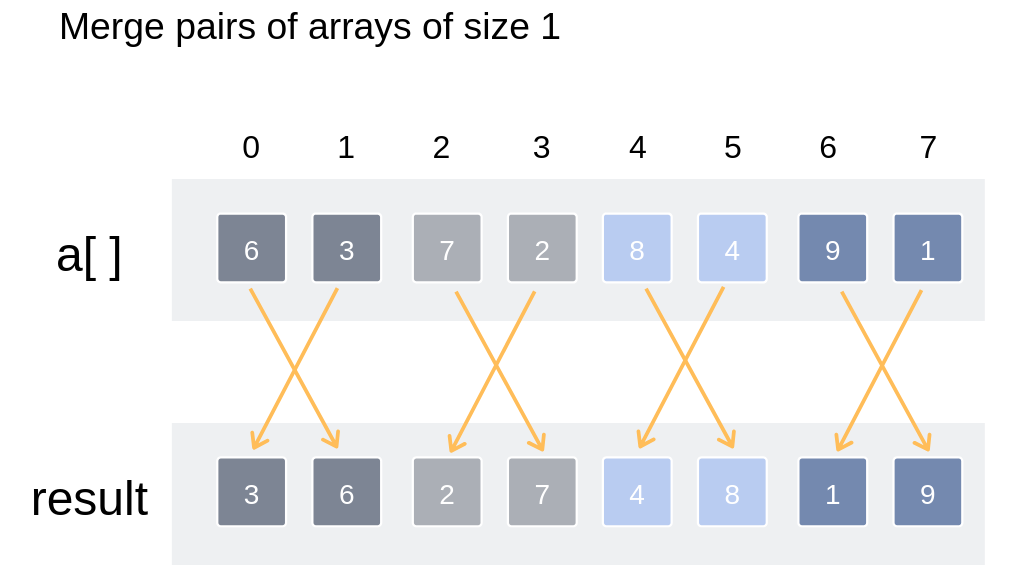Merge pairs of arrays of size 1

2. Iteration (2)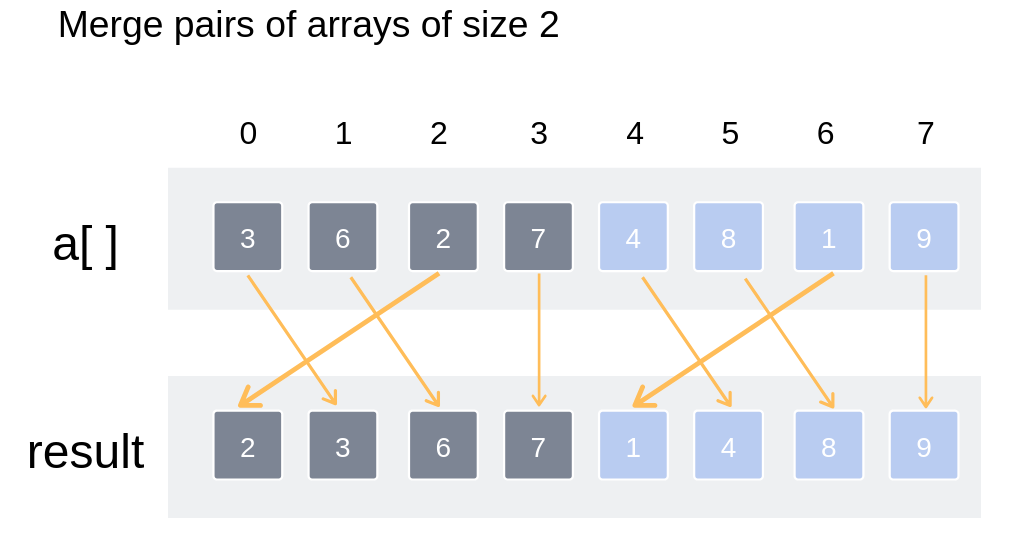Merge pairs of arrays of size 2

3. Iteration (3)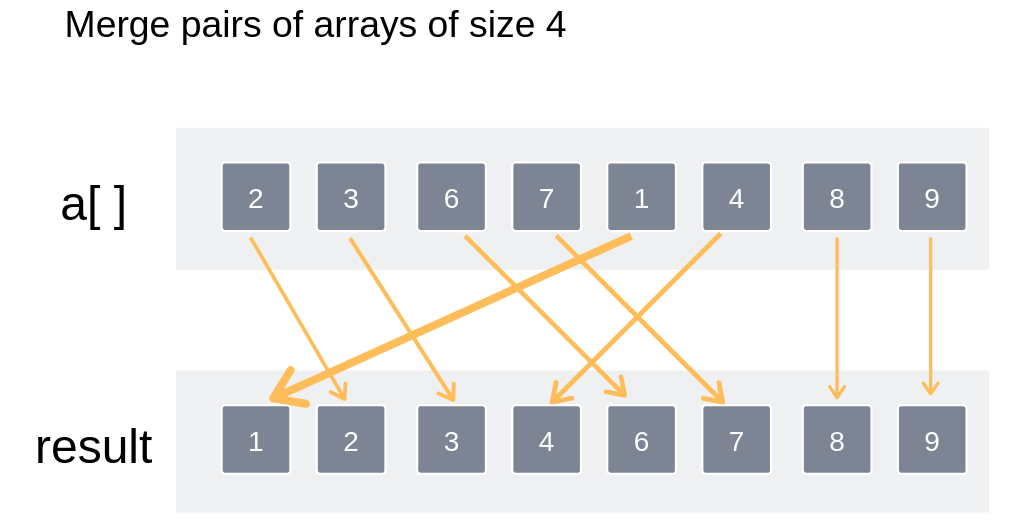Merge pairs of arrays of size 4

Thus the entire array has been sorted and merged.

## Serious about Learning Programming ?

Learn this and a lot more with Scaler Academy's industry vetted curriculum which covers Data Structures & Algorithms in depth.
Primers
Examples

## Arrays Problems

Array math
Value ranges
Problem Score Companies Time Status
Max Min 150
17:31
Merge Intervals 225 78:57
Merge Overlapping Intervals 225 48:24
Simulation array
Problem Score Companies Time Status
Perfect Peak of Array 200 48:49
Kth Row of Pascal's Triangle 225
28:32
Spiral Order Matrix II 225 48:40
Pascal Triangle 225 26:46
Anti Diagonals 225 41:46
Bucketing
Problem Score Companies Time Status
Triplets with Sum between given range 200
76:05
Balance Array 200 61:31
Find Duplicate in Array 450 40:13
Maximum Consecutive Gap 450 58:46
Arrangement
Problem Score Companies Time Status
Sort array with squares! 200 31:16
Largest Number 225 70:26
Rotate Matrix 300 60:26
Next Permutation 300 63:13
Find Permutation 300 56:00
Sorting
Problem Score Companies Time Status
Noble Integer 200 43:30
Wave Array 225 22:08
Hotel Bookings Possible 225 66:06
Max Distance 250 68:14
Maximum Unsorted Subarray 250 68:52
Space recycle
Problem Score Companies Time Status
Set Matrix Zeros 300 48:04
First Missing Integer 300 64:38
Maximum Sum Square SubMatrix 300 56:24
Missing / repeated number
Problem Score Companies Time Status
First Missing Integer 300 64:38
Repeat and Missing Number Array 350 63:55
N/3 Repeat Number 600
68:22

Problem Score Companies Time Status
Reorder Data in Log Files 200 42:21
Move Zeroes 200 24:24
Make equal elements Array 200 28:17
Segregate 0s and 1s in an array 200 14:14
Array Sum 200 30:37
Set Intersection 200 60:52
Occurence of Each Number 200 22:32
Greater of Lesser 200 12:03
Spiral Matrix 200 30:23
Product of All 200 29:54
Chips Factory 200 17:43
Greater than All 150 17:04
Pythagorean Triplets 200 22:32
Diagonal Flip 200 16:43
Positive Negative 100 9:33Free Mock Assessment
Help us know you better for the best experience
Current Employer *
Enter company name